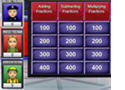# Math Games

• This is a fast-paced basketball game about multiplying decimals. Students can play it alone, with another friend, or even in teams.Decimal Jeopardy Game - This game is a fun way to review basic operations with decimals. It has a single-player mode as well as a multi-player feature. Students can play this game at home or at school as a review activity.2D Shapes Game (Concentration)
In this game students click on two cards to match the figure of a two-dimensional shape with its name. If there is a match, the problems remain on the page; if not, the cards are turned over.

This is an online word search game about place value vocabulary words.

In this game, students will solve word problems about money. The game has a virtual cash register that students will use to solve the problems

Students can play this game to assess their knowledge about factors and divisibility. It can be used as a review activity in the classroom or at home.

The questions in this jeopardy game focus on important concepts such as factors, multiples, prime factorization, GCF, and LCM.

In this game 5th grade students will practice reducing fractions to the simplest form.

This is another game about simplifying fractions. Students will have to first hit a homerun to be able to see and answer a math problem.

This online jeopardy game is is a fun way to review the four operations with fractions: addition, subtraction, multiplication, and division.Football Math Game - Adding Fractions In this game, students will try to pass the ball to the receiver. For each good pass, they will get to answer a math problem about adding fractions. For each correct answer they will earn 7 points, but each wrong answer will cost them 3 points.This millionaire game can be played in one or two teams. Students will practice changing decimals and fractions to percents.

In this online jeopardy game students will practice converting fractions to decimals and percents and vice-versa.

Get ready to have some fun multiplying fractions when you play this exciting soccer math game.

In this online millionaire game, 5th grade students will practice multiplying fractions while earning lots of points.Algebraic Expressions Millionaire Game This millionaire game can be played alone or in two teams. Students will have to identify the correct mathematical expressions that model given word expressions.